# Thread: Integrate (ln(x))^2/x^2

1. ## Integrate (ln(x))^2/x^2

Good afternoon,

I am hoping someone can give me a nudge in the right direction. I have the following problem:

$\displaystyle \int{\frac{ln(x)^2dx}{x^2}}$

First use substitution, then use integration by parts.

What is the proper way to handle this via substitution?

$\displaystyle u = ln(x) , du = \frac{1}{x}$ ?

$\displaystyle u = ln(x)^2 , du = \frac{1}{x^2}$ ?

2. Why not do parts right away (twice) - integrate 1/x^2 and differentiate ln^2(x) (with the chain rule). Pic in a mo

btw for $\displaystyle u = \ln^2(x)$, $\displaystyle du = 2\ln(x)\ \frac{1}{x}$

Edit: excuse the delay, anyway, just in case a picture helps...... where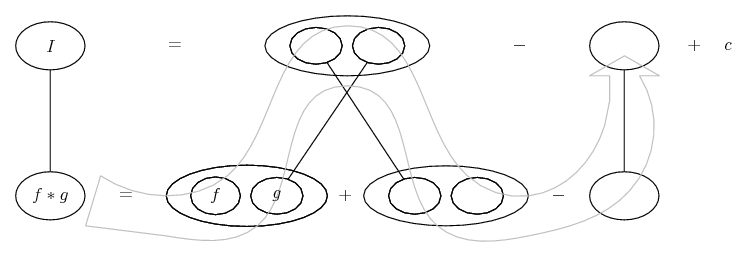... is lazy integration by parts, doing without u and v, and ...... is the product rule, straight lines differentiating downwards (integrating up) with respect to x.

Full size:
Spoiler: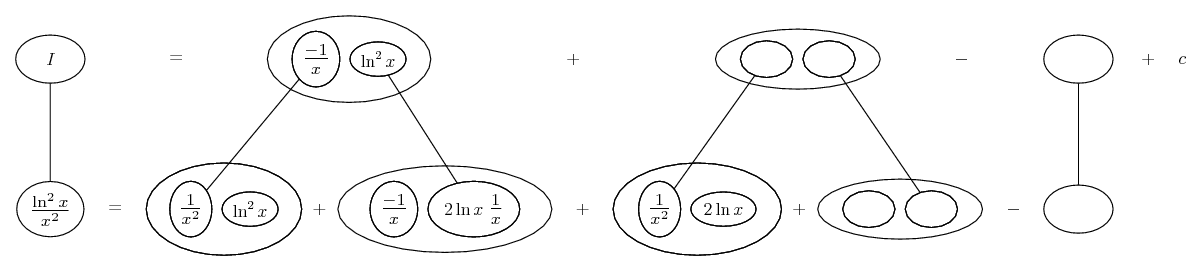Zooming in on the chain rule (in a jiff)...Key and full-size:
Spoiler: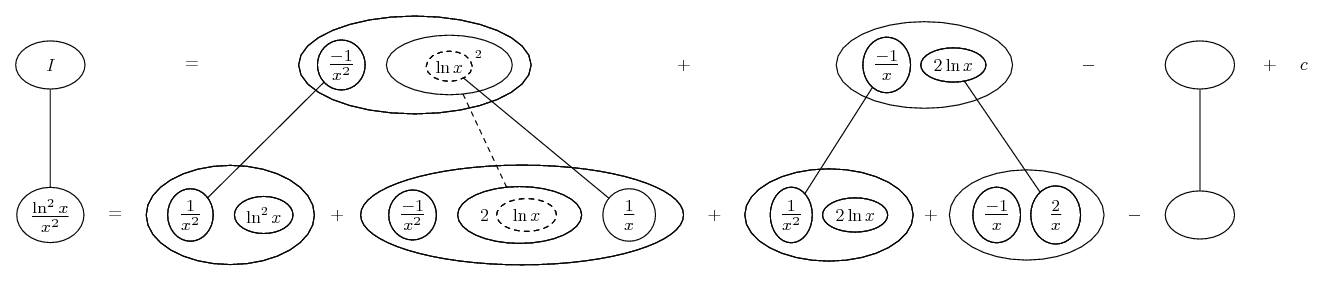... is the chain rule. Straight continuous lines still differentiate downwards (integrate up) with respect to x, and the straight dashed line similarly but with respect to the dashed balloon expression (the inner function of the composite which is subject to the chain rule).
Spoiler: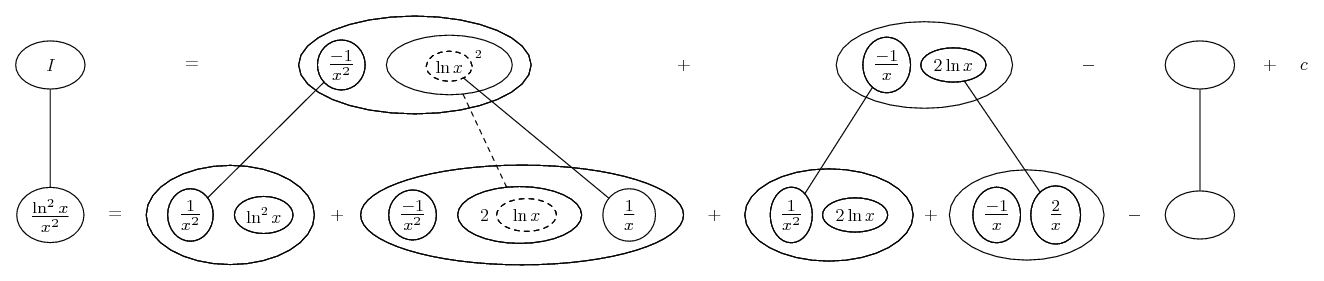Spoiler: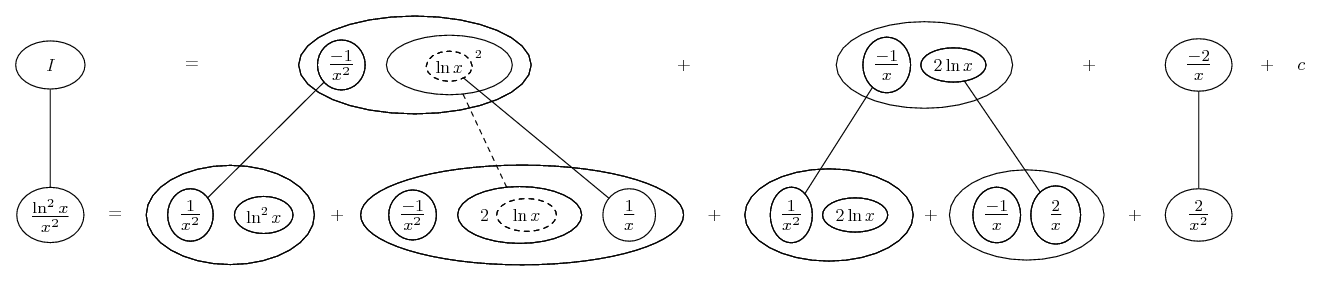I'll look at the subbing first, also.
_________________________________________

Don't integrate - balloontegrate!

Balloon Calculus; standard integrals, derivatives and methods

Balloon Calculus Drawing with LaTeX and Asymptote!

3. Ah, that right...

$\displaystyle \frac{d}{dx}ln(x) = \frac{d}{dx}ln(x)*ln(x)$

The problem states to apply substitution first, so I am trying to do that...

4. Subbing u = ln x and du = 1/x gives

$\displaystyle \int \frac{u^2}{e^u} du$

which is also suitable for parts...Full:
Spoiler: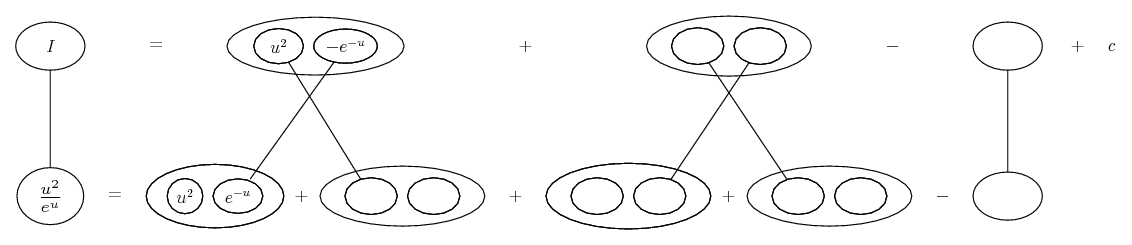Spoiler: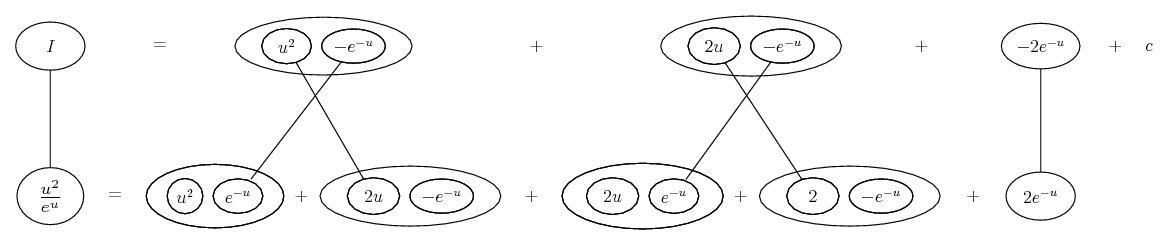Then back-substitute, of course. Here is roughly how I would show the u-sub and the parts in one pic.

_________________________________________

Don't integrate - balloontegrate!

Balloon Calculus; standard integrals, derivatives and methods

Balloon Calculus Drawing with LaTeX and Asymptote!

5. I just use ILATE for by-parts integration:

I = inverse trig
L = logarithmic
A = algebraic
T = trig
E = exponential.

Whichever function is higher on the list gets to be u, and whichever is lower on the list gets to be dv.

6. This is embarassing, but this is simply not "clicking" for me. Everything up until this point has come back to me in an "ah hah" sort of fashion. However, I am stuck on the simple act of substitution.

Would someone mind walking me through the act of selecting the proper "u" and "du" for this equation? After that, I think I can solve by parts.

Thank you.

7.Originally Posted by tom@ballooncalculusSubbing u = ln x and du = 1/x gives

$\displaystyle \int \frac{u^2}{e^u} du$
This is the part I didn't do as a diagram - so I'm hoping the fact that you're struggling with it justifies one now. (Or in a minute.) However, I should really have written

Subbing u = ln x and du = 1/x dx

and then...

$\displaystyle \int \frac{\ln^2 x}{x^2}\ dx$

$\displaystyle =\ \int \frac{\ln^2 x}{x}\ \frac{1}{x}\ dx$

$\displaystyle =\ \int \frac{u^2}{e^u}\ du$

Just in case a picture helps...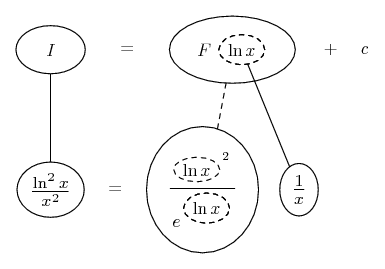The general drift...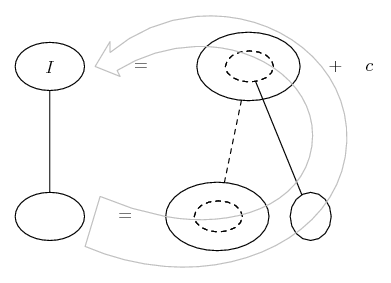As above, straight continuous lines differentiate downwards (integrate up) with respect to x, and the straight dashed line similarly but with respect to the dashed balloon expression (the inner function of the composite which is subject to the chain rule).

So, F is an anti-derivative of the big lower balloon with respect to the dashed balloon - which of course we find it convenient to call u, hence...

$\displaystyle \int \frac{u^2}{e^u}\ du$

_________________________________________

Don't integrate - balloontegrate!

Balloon Calculus; standard integrals, derivatives and methods

Balloon Calculus Drawing with LaTeX and Asymptote!

8.Originally Posted by MechEngThis is embarassing, but this is simply not "clicking" for me.
Hey, perhaps the numerator $\displaystyle \ln{x}^2$ instead of $\displaystyle \ln^2(x)$, which is little easier and probably more common?
In any case, it can't get clearer than Tom's posts, so try to follow them. I don't think there is a way
to solve this using just substitution without by parts.

9. I just had theat "click" i was referring to. Thanks guys!

,

,

,

,

,

,

,

,

,

,

,

,

,

,

# (lnx)^2/x^2 적분

Click on a term to search for related topics.

#### Search Tags

integrate, lnx2 or x2